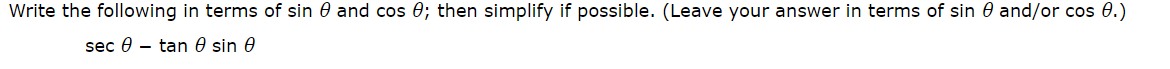Write the following in terms of sin 0 and cos 0; then simplify if possible. (Leave your answer in terms of sin 0 and/or cos 0.)sec - tan 0 sin e

Question

practice question, I attached a photohelp_outlineImage TranscriptioncloseWrite the following in terms of sin 0 and cos 0; then simplify if possible. (Leave your answer in terms of sin 0 and/or cos 0.) sec - tan 0 sin e fullscreen
Step 1

Simplify the given expression in terms of Sin θ, Cos θ

Step 2

The values of secθ  and tanθ  in...

Want to see the full answer?

See Solution

Want to see this answer and more?

Our solutions are written by experts, many with advanced degrees, and available 24/7

See Solution
Tagged in

Trigonometric Ratios